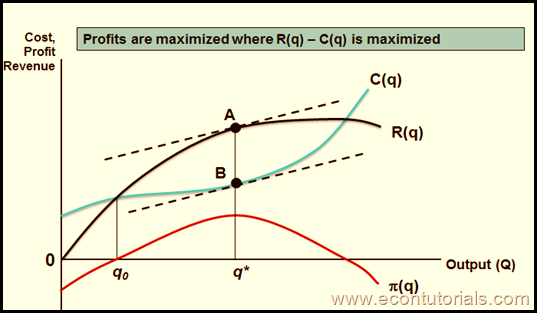Select Page

There is a very basic concept of understanding Profit maximization either for Perfect Competition or another market model. For almost all markets, the concept is similar.

## Total Revenue

If Q is output of the firm, Total Revenue is :

• Total Revenue = Price x Quantity
• TR=P*Q

## Profit

• Profit (PIE)= Total Revenue – Total Cost
• P=TR-TC

So a profit for a firm is the difference between the Revenue it receives and the cost it incurs during the production process. Which also means that firms chooses output at that point when revenue and costs are at its maximum and minimum point respectively. Graphically the greatest distance between the two shows the Profit level.

Do not forget that when discussing revenue, we have already described in our previous lectures that if a firm wants to sell more of their  product quantity requires a reduction in their Price . And if the firm or producer tries to raise price (especially incase of perfect competition, the sales will become zero)

• At  the Beginning, fixed and variable costs are too large and Revenue is Zero. So profits will be negative. Think about any business that you decide to start. Almost in every business you are stuck with initial investment and do not expect to have revenue at the beginning.
• As output begins to rise, revenue gradually increases more than the rise in costs.
• For every market model, Profits is maximized at the point where MR=MC.
• We can also say that profits are maximized at the point where the Slope of Revenue Curve (MR) equals the Slope of Cost Curve (MC).
• It is a point after which there wont be any effect over the revenue even if the output increases.

## Perfect Competition Graphically(Upnext: When and How to choose an output in Perfect Competition Short Run Period)

Shares